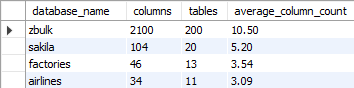## Find number of columns in MariaDB database

Article for:

The query returns basic statistics of the number of columns in a database (schema).

## Query

``````select database_name,
columns,
tables,
cast((1.0*columns/tables) as decimal(10,2)) as average_column_count
from (
select cols.table_schema as database_name,
count(*) as 'columns',
count(distinct cols.table_name) as 'tables'
from information_schema.columns as cols
inner join information_schema.tables as tabs
on tabs.table_schema = cols.table_schema
and tabs.table_name = cols.table_name
and table_type = 'BASE TABLE'
and tabs.table_schema not in ('mysql', 'sys', 'information_schema',
'performance_schema')
group by database_name
) as q
order by columns desc;
``````

## Columns

• columns - total number of columns in a database (schema)
• tables - number of tables in a database (schema)
• average_column_count - average number of columns per table in a database (schema)

## Rows

• One row: represents a database (schema)
• Scope of rows: all databases (schemas)
• Ordered by: number of columns in descending order

## Sample results0